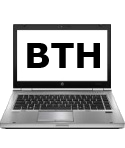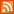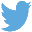Bucaro TecHelpHTTPS Encryption not required because no account numbers orpersonal information is ever requested or accepted by this siteCustom Search# Computer Number Systems Made Simple

Digital computers perform all of their amazing work with only two voltage levels. One of those voltages is 0 volts. The other voltage can be anything from 2.5 volts or lower, up to 5 volts, as long as it's not 0 volts. A computer interprets 0 volts as "0", and the other voltage as "1".

Humans use a decimal based number system. The decimal numbering system uses the ten characters 0,1,2,3,4,5,6,7,8, and 9 to represent numbers. That's why decimal numbers are sometimes called base-10 numbers.

The binary system used by computers has only two characters; 0 and 1. The binary numbers are base-2 numbers. You would think that would make computers very limited, but their amazing speed more than compensates for the limited number of characters in their number system.

The decimal system is a positional system. That means the actual value of a digit depends on its position in a number. For example in the number 10, the 1 represents ten units, while the number 100 represents one hundred units. To interpret a number you add together the values of all the digits in their positions in the number. For example 110 equals one hundred and ten units.

The binary system is also a positional system. The binary number 10 equals decimal system 2. The binary number 100 equals decimal system 4. Similar to the decimal system, to interpret a number you add together the values the digits in their positions. For example the binary number 110 equals decimal system 6.

You might think it's very difficult to convert binary to decimal but all you have to do is think in powers of 2. Lets think about binary numbers with 4 characters. Going from right to left the powers of 3 would be valued at decimal 1, 2, 4, and 8. It's actually easier to think from left to right, so the positions would be valued at 8,4,2,1.

So a the binary number 1010 equals 8+2 or decimal 10. The binary number 0110 equals 4+2 or decimal 6. The binary number 1101 equals 8+4+1 or decimal 13.

Computers today use 64 bit, or 128 bit, or even larger numbers. You can imagine this would make them very difficult to convert to decimal, or even to comprehend at all. To allow humans to more easily comprehend binary numbers, the hexadecimal number system is used.

The hexadecimal number system base-16. That means it uses 16 different characters to represent numbers. For numbers up to 9, the decimal system and the hexadecimal system use the same characters. But after 9, the decimal system runs out of characters. The hexadecimal continues on by using the characters a, b, c, d, e, and f to complete the required 16 character set.

So counting in hexadecimal goes like this; 0,1,2,3,4,5,6,7,8,9,a,b,c,d,e,f. The hexadecimal system is also a positional system. So you could continue counting like this; 10, 11, 12, 13, 14, 15, 16, 17, 18, 19, 1a, 1b, 1c, 1d, 1e, 1f, then 20.

When you get up to 9f, the next hexadecimal number would be a0.

It's very important to know if you're looking at a decimal number or a hexadecimal number, because the hexadecimal number 20 mentioned above is actually equal to 32 in the decimal system.

You might think it would be a problem converting hexadecimal numbers to decimal numbers, but in actuality this is rarely done. Hexadecimal numbers are used extensively in computers and networking, and after using them for a while, they become just as intuitive as decimal numbers. In other words, you can think about and use hexadecimal numbers all day long without ever desiring to convert them to decimal.

About the only thing you need to memorize about hexadecimal numbers is the maximum number of items that will fit in a specific number of hexadecimal characters. In other words, hexadecimal f represents 16 different states. This might be confusing because hexadecimal f equals 15 decimal, but in computers, the number 0 actually means something. It means the zero-ith item. In other words, computers start counting with 0, not with 1 like in normal decimal counting.

Following the same thinking, hexadecimal ff represents 256 decimal different states. Hexadecimal fff represents 4096 different states. Hexadecimal ffff represents 65,536 different states.

That last one is important because the latest revision of the Internet Protocol (IPv6) uses a 128-bit addresses represented as eight groups of four hexadecimal digits separated by colons, called octets. For example 2001:0db8:0000:0000:0000:ff00:0042:8329 is an IPv6 address. But to make things confusing, in actuality this address would be expressed as: 2001:db8::ff00:42:8329.

This confusing number is created by removing all leading zeros, for example :0042: becomes :42: and by omitting consecutive sections of zeroes, for example :0000: becomes :: . Fortunately, it will be a while before IPv6 can be fully implemented.

As a prospective computer networking technician, I would recommend playing around with converting a few numbers back and forth between binary, decimal, and hexadecimal. But as a professional networking technician you'll need to intuitively understand and use hexadecimal numbers without ever converting them to any other system.

 .menubtn { margin-top:10px; margin-left:50px; width:220px; opacity:1; border-style:solid; border-color:#009000; background-color:#66ff66; transition-property: opacity; transition-duration: 1s; transition-timing-function: ease-out; } .menubtn:hover { opacity:1; background-color:#e5ff23; } Menu - More Networking BasicsRSS FeedFollow @Stephen Bucaro Computer Networking Sections[Site User Agreement] [Privacy Policy] [Site map] [Search This Site] [Contact Form]
Copyright©2001-2021 Bucaro TecHelp 13771 N Fountain Hills Blvd Suite 114-248 Fountain Hills, AZ 85268